## How to Calculate and Solve for Wavelength | Optical Properties

The wavelength is illustrated by the image below.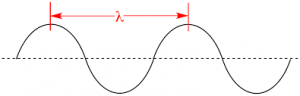To compute for wavelength, three essential parameters are needed and these parameters are Planck’s Constant (h), Speed of Light (c) and Energy Gap (Eg).

The formula for calculating wavelength:

λ = hc/Eg

Where:

λ = Wavelength (Eg)
h = Planck’s Constant
c = Speed of Light
Eg = Energy Gap

Given an example;
Find the wavelength when the planck’s constant is 6.626E-24, the speed of light is 3E8 and the energy gap is 24.

This implies that;

h = Planck’s Constant = 6.626E-24
c = Speed of Light = 3E8
Eg = Energy Gap = 24

λ = hc/Eg
λ = (6.62607004e-24)(300000000)/24
λ = 1.987821012e-15/24
λ = 8.28e-17

Therefore, the wavelength is 8.28e-17m.

## How to Calculate and Solve for Energy of Electromagnetic Radiation | Optical Properties

The energy of electromagnetic radiation is illustrated by the image below.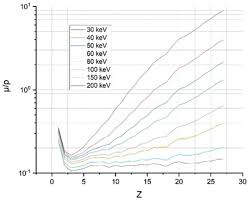To compute for energy of electromagnetic radiation, two essential parameters are needed and these parameters are Planck’s Constant (h) and Frequency (f).

The formula for calculating the energy of electromagnetic radiation:

E = hf

Where:

E = Energy of Electromagnetic Radiation
h = Planck’s Constant
f = Frequency

Given an example;
Find the energy of electromagnetic radiation when the planck’s constant is 6.62607004e-34 and the frequency is 20.

This implies that;

h = Planck’s Constant = 6.62607004e-34
f = Frequency = 20

E = hf
E = (6.62607004e-34)(20)
E = 1.325214008e-32

Therefore, the energy of electromagnetic radiation is 1.325214008e-32 J.

## How to Calculate and Solve for Eyring Equation for Predicting Area | Transport Phenomena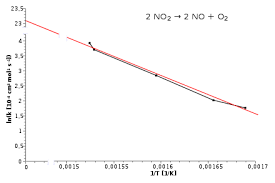The image above represents eyring equation for predicting area.

To compute for eyring equation for predicting area, three essential parameters are needed and these parameters are Avogadro’s Number (NA), Planck’s Constant (h) and Molar Volume (V).

The formula for calculating eyring equation for predicting area:

A ≋ NAh / V

Where:

A = Eyring Equation for Predicting Area
h = Planck’s Constant
V = Molar Volume

Let’s solve an example;
Find the eyring equation for predicting area when the avogadro’s number is 6.023e+23. the planck’s constant is 6.626e-34 and the molar volume is 15.

This implies that;

NA = Avogadro’s Number = 6.023e+23
h = Planck’s Constant = 6.626e-34
V = Molar Volume = 15

A = NAh / V
A = (6.023e+23)(6.626e-34) / 15
A = 3.99e-10 / 15
A = 2.66e-11

Therefore, the eyring equation for predicting area is 2.66e-11 m².

## How to Calculate and Solve for Stefan Boltzmann’s Constant | Radiation Heat Transfer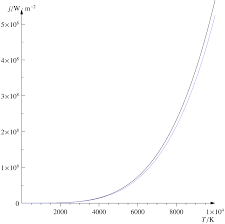The image above represents stefan boltzmann’s constant.

To compute for stefan boltzmann’s constant, three essential parameters are needed and these parameters are Boltzmann’s Constant (KB), Planck’s Constant (h) and Speed of Light (c).

The formula for calculating stefan boltzmann’s constant:

σ = 2π5KB4 / 15c²h5

Where:

σ = Stefan Boltzmann’s Constant
KB = Boltzmann’s Constant
h = Planck’s Constant
c = Speed of Light

Let’s solve an example;
Find the stefan boltzmann’s constant when the boltzmann’s constant is 42, the planck’s constant is 34 and the speed of light is 26.

This implies that;

KB = Boltzmann’s Constant = 42
h = Planck’s Constant = 34
c = Speed of Light = 26

σ = 2π5KB4 / 15c²h5
σ = 2π5(42)4 / 15(26)²(34)5
σ = 2(306.019)(3111696) / 15(676)(45435424)
σ = 1904480458.135 / 460715199360
σ = 0.00413

Therefore, the stefan boltzmann’s constant is 0.00413.

## How to Calculate and Solve for Monochromatic Emissive Power | Radiation Heat Transfer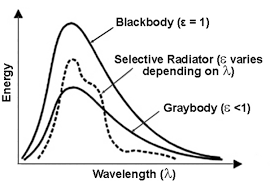The image above represents monochromatic emissive power.

To compute for monochromatic emissive power, five essential parameters are needed and these parameters are Planck’s Constant (h), Velocity of Light (c), Wavelength (λ), Boltzmann’s Constant (KB) and Temperature (T).

The formula for calculating monochromatic emissive power:

ebx = 2πhc²λ-5 / exp(ch/KBλT) – 1

Where:

ebx = Monochromatic Emissive Power | Planck’s Equation
h = Planck’s Constant
c = Velocity of Light
λ = Wavelength
KB = Boltzmann’s Constant
T = Temperature

Let’s solve an example;
Find the monochromatic emissive power when the planck’s constant is 6.626E-24, the velocity of light is 3E8, the wavelength is 22, the boltzmann’s constant is 1.380E-23 and the temperature is 10.

This implies that;

h = Planck’s Constant = 6.626E-24
c = Velocity of Light = 3E8
λ = Wavelength = 22
KB = Boltzmann’s Constant = 1.380E-23
T = Temperature = 10

ebx = 2πhc²λ-5 / exp(ch/KBλT) – 1
ebx = 2π(6.62607004e-34)(300000000)²(22)-5 / exp((300000000)(6.62607004e-34)/(1.38064852e-23)(22)(10)) – 1
ebx = 2π(6.62607004e-34)(90000000000000000)(1.94037e-7) / exp(1.987821012e-25/3.037426744e-21) – 1
ebx = 7.270512005456302e-23 / exp(0.00006544424539379114) – 1
ebx = 7.270512005456302e-23 / 1.0000654463869152 – 1
ebx = 7.270512005456302e-23 / 0.00006544638691519111
ebx = 1.11e-18

Therefore, the monochromatic emissive power is 1.11e-18.

## How to Calculate and Solve for Velocity | De Broglie’s Law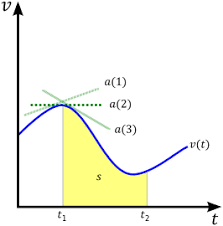The image above represents velocity.

To compute for velocity, three essential parameters are needed and these parameters are Planck’s Constant (h), Wavelength (λ) and Mass (m).

The formula for calculating velocity:

v = h / λm

Where;

v = velocity
λ = wavelength
h = Planck’s constant
m = mass

Let’s solve an example;
Find the velocity when the wavelength is 10, the planck’s constant is 6.626e-34 and the mass is 5.

This implies that;

λ = wavelength = 10
h = Planck’s constant = 6.626e-34
m = mass = 5

v = h / λm
v = 6.62607004e-34 (10)(5)
v = 6.62607004e-34 (50)
v = 1.33e-35

Therefore, the velocity is 1.33e-35 m/s.

## How to Calculate and Solve for Mass | De Broglie’s Law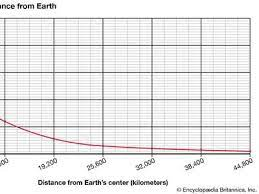The image above represents mass.

To compute for mass, three essential parameters are needed and these parameters are Planck’s Constant (h), Wavelength (λ) and Velocity (v).

The formula for calculating the mass:

m = h / λv

Where;

m = mass
λ = wavelength
h = Planck’s constant
v = velocity

Let’s solve an example;
Find the mass when the wavelength is 4, the planck’s constant is 6.626e-34 and the velocity is 2.

This implies that;

λ = wavelength = 4
h = Planck’s constant = 6.626e-34
v = velocity = 2

m = hλv
m = 6.62607004e-34(4)(2)
m = 6.62607004e-34(8)
m = 8.28e-35

Therefore, the mass is 8.28e-35 kg.

## How to Calculate and Solve for Wavelength | De Broglie’s Law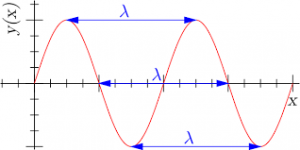The image above represents wavelength.

To compute for wavelength, three essential parameters are needed and these parameters are Planck’s Constant (h), Mass (m) and Velocity (v).

The formula for calculating wavelength:

λ = hmv

Where

λ = wavelength
h = Planck’s constant
m = mass
v = velocity

Let’s solve an example;
Find the wavelength when the planck’s constant is 6.62e-34, the mass is 21 and the velocity is 11.

This implies that;

h = Planck’s constant = 6.62e-34
m = mass = 21
v = velocity = 11

λ = hmv
λ = 6.62607004e-34(21)(11)
λ = 6.62607004e-34(231)
λ = 2.86e-36

Therefore, the wavelength is 2.86e-36 m.

Calculating the Mass when the Wavelength, the Planck’s Constant and the Velocity is Given.

m = h / λv

Where;

m = mass
λ = wavelength
h = Planck’s constant
v = velocity

Let’s solve an example;
Find the mass when the wavelength is 18, the planck’s constant is 6.626e-34 and the velocity is 6.

This implies that;

λ = wavelength = 18
h = Planck’s constant = 6.626e-34
v = velocity = 6

m = h / λv
m = 18 / 6.626e-34 x 6
m = 18 / 3.976e-33
m = 4.53

Therefore, the mass is 4.53 m.